### Notes on Bias of Estimators

Generalized linear mixed models are nonlinear models, and the estimation techniques rely on approximations to the log likelihood or approximations of the model. It is thus not surprising that the estimates of the covariance parameters and the fixed effects are usually not unbiased. Whenever estimates are biased, questions arise about the magnitude of the bias, its dependence on other model quantities, and the order of the bias. The order is important because it determines how quickly the bias vanishes while some aspect of the data increases. Typically, studies of asymptotic properties in models for hierarchical data suppose that the number of subjects (clusters) tends to infinity while the size of the clusters is held constant or grows at a particular rate. Note that asymptotic results so established do not extend to designs with fully crossed random effects, for example.

The following paragraphs summarize some important findings from the literature regarding the bias in covariance parameter and fixed-effects estimates with pseudo-likelihood, Laplace, and adaptive quadrature methods. The remarks draw in particular on results in Breslow and Lin (1995), Lin and Breslow (1996), and Pinheiro and Chao (2006). Breslow and Lin (1995) and Lin and Breslow (1996) study the "worst case" scenario of binary responses in a matched-pairs design. Their models have a variance component structure, comprising either a single variance component (a subject-specific random intercept; Breslow and Lin 1995) or a diagonal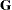matrix (Lin and Breslow 1996). They study the bias in the estimates of the fixed-effectsand the covariance parameters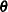when the variance components are near the origin and for a canonical link function.

The matched-pairs design gives rise to a generalized linear mixed model with a cluster (subject) size of 2. Recall that the pseudo-likelihood methods rely on a linearization and a probabilistic assumption that the pseudo-data so obtained follow a normal linear mixed model. Obviously, it is difficult to imagine how the subject-specific (conditional) distribution would follow a normal linear mixed models with binary data in a cluster size of 2. The bias in the pseudo-likelihood estimator ofis of order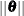. The bias for the Laplace estimator ofis of smaller magnitude; its asymptotic bias has order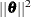.

The Laplace methods and the pseudo-likelihood method produce biased estimators of the variance component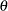for the model considered in Breslow and Lin (1995). The order of the asymptotic bias for both estimation methods is, asapproaches zero. Breslow and Lin (1995) comment on the fact that even with matched pairs, the bias vanishes very quickly in the binomial setting. If the conditional mean in the two groups is equal to
, then the asymptotic bias factor of the pseudo-likelihood estimator is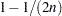, whereis the binomial denominator. This term goes to 1 quickly asincreases. This result underlines the importance of grouping binary observations into binomial responses whenever possible.

The results of Breslow and Lin (1995) and Lin and Breslow (1996) are echoed in the simulation study in Pinheiro and Chao (2006). These authors also consider adaptive quadrature in models with nested, hierarchical, random effects and show that adaptive quadrature with a sufficient number of nodes leads to nearly unbiased—or least biased—estimates. Their results also show that results for binary data cannot so easily be ported to other distributions. Even with a cluster size of 2, the pseudo-likelihood estimates of fixed effects and covariance parameters are virtually unbiased in their simulation of a Poisson GLMM. Breslow and Lin (1995) and Lin and Breslow (1996) "eschew" the residual PL version (METHOD=RSPL) over the maximum likelihood form (METHOD=MSPL). Pinheiro and Chao (2006) consider both forms in their simulation study. As expected, the residual form shows less bias than the MSPL form, for the same reasons REML estimation leads to less biased estimates compared to ML estimation in linear mixed models. The gain is modest, however; see, for example, Table 1 in Pinheiro and Chao (2006). When the variance components are small, there is a sufficient number of observations per cluster, and a reasonable number of clusters, then pseudo-likelihood methods for binary data are very useful—they provide a computational expedient alternative to numerical integration, and they allow the incorporation of R-side covariance structure into the model. Because many group randomized trials involve many observations per group and small random effects variances, Murray et al. (2004) term questioning the use of conditional models for trials with binary outcome an "overreaction."